Start by making sure you're looking at your smart meter on the wall and not an in-home displayIf you think your smart meter isn't shown below, please send us a clear photograph showing the entire front of your meter. We'll find out how to read your meter and we can add your meter type to our guide.

Once you can read your meter, submitting readings regularly will keep you on top of your energy. You can set up email reminders.

## Electricity smart meters

To find your electricity read you're looking for a number followed by "KWH" (but remembering to ignore the numbers after the decimal place).

### If you have a keypad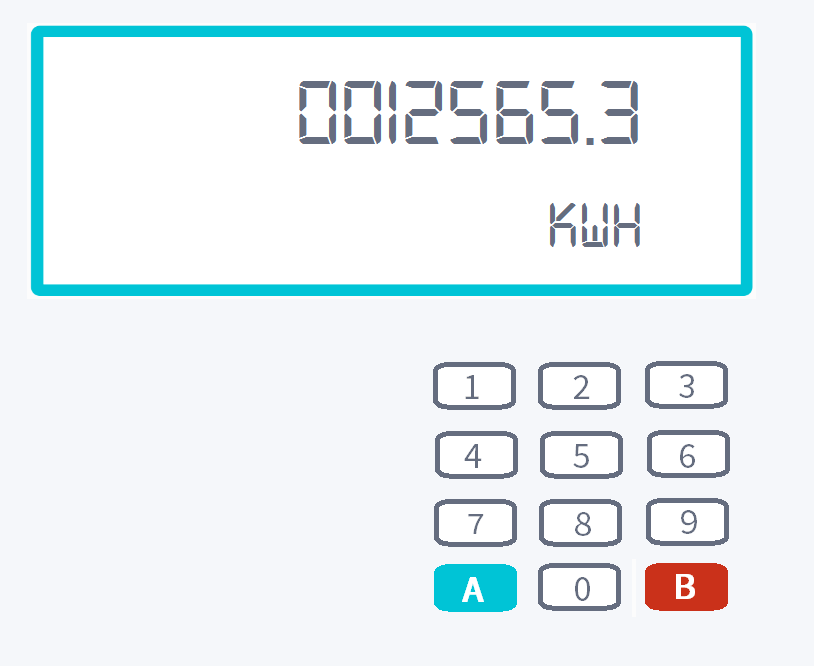To get a reading from these smart meters:

1. Press 9 on the keypad.
2. A series of letters will appear: IMP KWH.
3. Then you’ll see 8 digits (e.g. 0012565.3) followed by kWh at the bottom right of the screen.

If you have an Economy 7 smart meter (your meter records day and night usage):

1. Press 6 on the keypad until you see 'IMP R01' followed by 8 digits - this is usually your night/off-peak reading.
2. Then keep on pressing 6 on the keypad until you see 'IMP R02' followed by 8 digits - this is usually your peak/normal reading.
3. Ignore any digits after a decimal point if you have one. We only require the whole numbers.

### If you have two buttons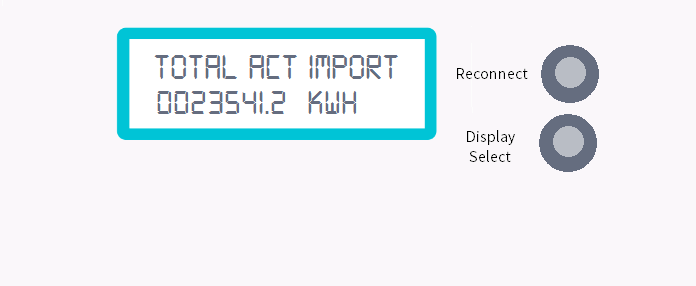To get a reading from these smart meters:

1. Press the "Display" or "Display Select" button.
2. By pressing the button the meter display will scroll through to the meter reading. The number you're after is the one followed by kWh.
3. As before write down all the digits, ignoring any digits after a decimal point if you have one. We only require the whole numbers.
4. In this example the meter reading is 23541.

### If you have A and B buttons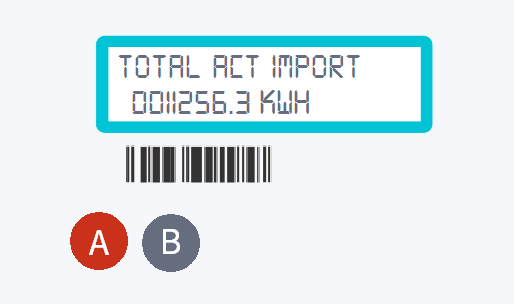To get a reading from these smart meters:

1. Press the A button.
2. Keep on pressing this until you get to TOTAL ACT IMPORT.
4. Ignore any digits after a decimal point if you have one. We only require the whole numbers.
5. In this example the meter reading is 11256.

### If you have 3 buttons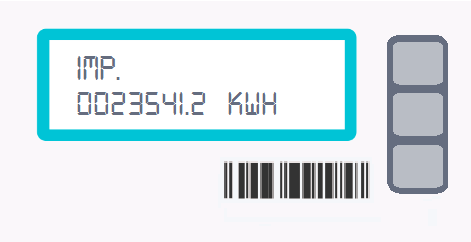To get a reading from these smart meters:

1. Press the middle button.
3. If you have a 2-rate meter, R1 indicates Rate 1, R2 is Rate 2 and T is total.
4. You can press the middle button again to see all the readings.
5. Ignore any digits after a decimal point if you have one. We only require the whole numbers.
6. In this example the meter reading is 23541.

### If you have an orange button on the left and blue buttons on the right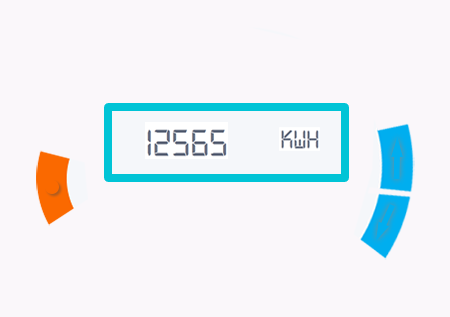To get a reading from these smart meters:

1. Press the orange button.
2. You'll see a row of digits followed by kWh.
3. Ignore any digits after a decimal point if you have one. We only require the whole numbers.
4. The reading in this example is 12565.

## Gas smart meters

To find your gas read you're looking for the number followed by "M3" (but remembering to ignore the numbers after the decimal place).

### If you have a keypad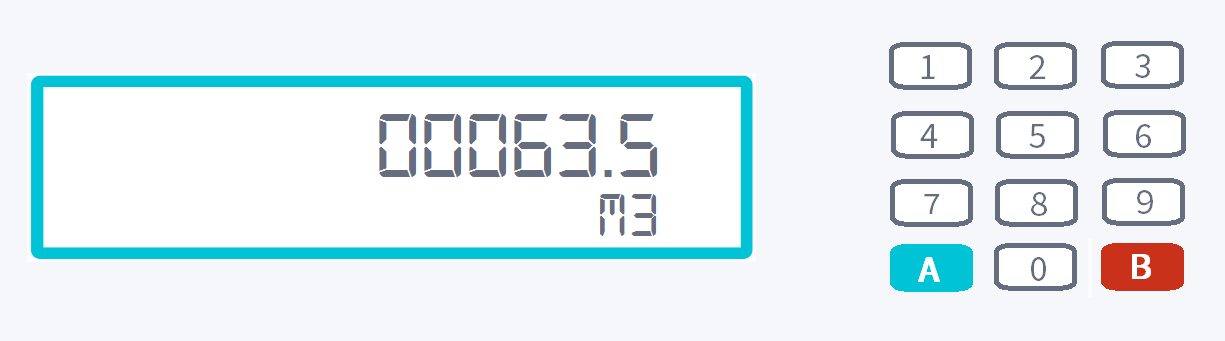To get a reading from these smart meters:

1. Press 9 on the keypad.
2. VOLUME will appear on the screen.
3. Then you’ll see a row of digits (e.g. 00063.5) followed by M3 at the bottom right of the screen.
4. Ignore any digits after a decimal point if you have one. We only require the whole numbers.
5. The read in this example is 00063.

### If you have A and B buttons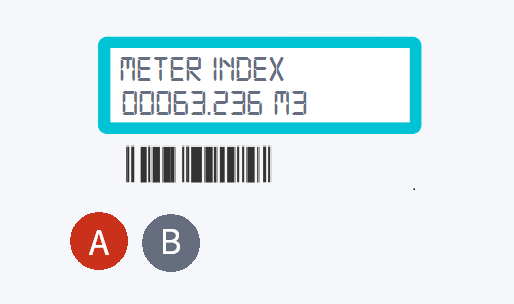To get a reading from these smart meters:

1. Press the A button.
2. Keep on pressing this until you get to METER INDEX.
4. Ignore any digits after a decimal point if you have one. We only require the whole numbers.
5. In this example the meter reading is 63.

### If you have a left arrow, a circle and a right arrow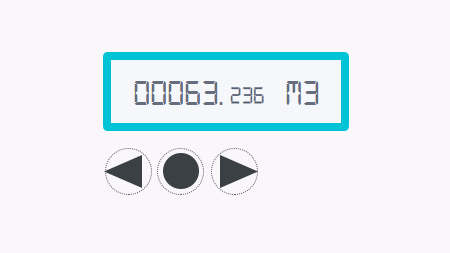To get a reading from these smart meters:

1. Press the middle 'circle' button.
2. The screen will show 'current meter reading' written along the top.
3. You'll see a row of digits followed by m3.
4. Ignore any digits after a decimal point if you have one. We only require the whole numbers.
5. The read in this example is 63.

### If you have 3 square red buttons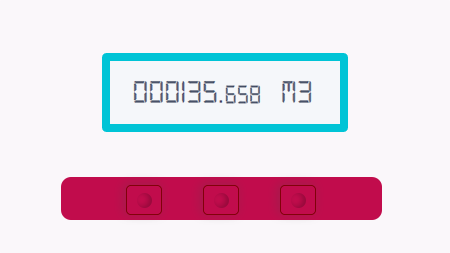or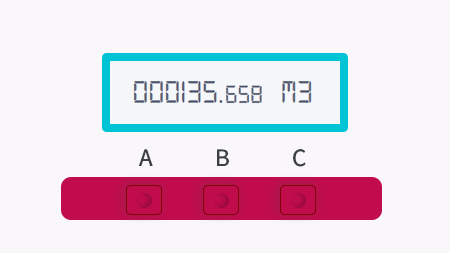Your buttons may or may not be labelled A, B and C. To get a reading from these smart meters:

1. Press the 'A' or left button.
2. You'll see a row of digits followed by m3.
3. Ignore any digits after a decimal point if you have one. We only require the whole numbers.
4. The read in this example is 135.

### If you have an orange and a blue arrow to the right of the screen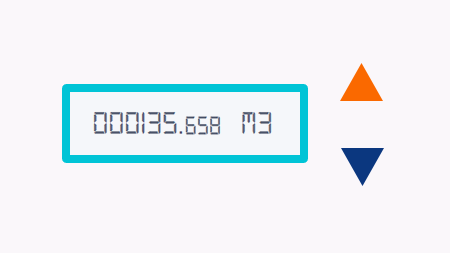To get a reading from these smart meters:

1. Press the orange button.
2. You'll see a row of digits followed by m3.
3. Ignore any digits after a decimal point if you have one. We only require the whole numbers.
4. In this example the meter reading is 135.

### If you have two white buttons to the right of your display. Your buttons might have arrows and other markings around them.To get a reading from these smart meters:

1. Press the right button. This might be labelled 'OK'.
2. The display should wake up and you'll see a row of digits followed by m3.
3. Ignore any digits after a decimal point if you have one. We only require the whole numbers.
4. In this example the meter reading is 135.